# Introduction to Functional Programming with Vavr

Pure functions, High Order functions, Immutability, Composition, Currying, Recursion, Lazy, Functors, Foldable, Applicative, Category, Semi Group, Monoid, Monad.### Concepts

I encourage you to follow it, but for this article I will take some of its examples and just show you how you can do it in Java with Vavr.

All images are courtesy of Aditya Bhargava, the author of the article above.

#### Functors

When a value is wrapped in a box, you can’t apply a normal function to it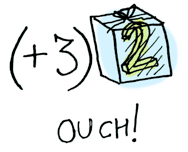This is where map comes in. map knows how to apply functions to values that are wrapped in a box.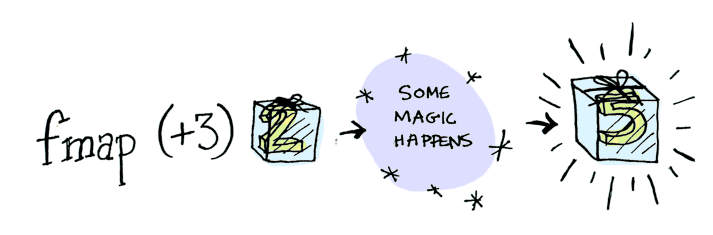``````Option.of(2).map(i -> i + 3); // Some(5)
Option.<Integer>of(null).map(i -> i + 5); // None``````

A functor is any type that defines how map works.

Here’s what is happening behind the scenes when we write `Option.of(2).map(i -> i + 3)`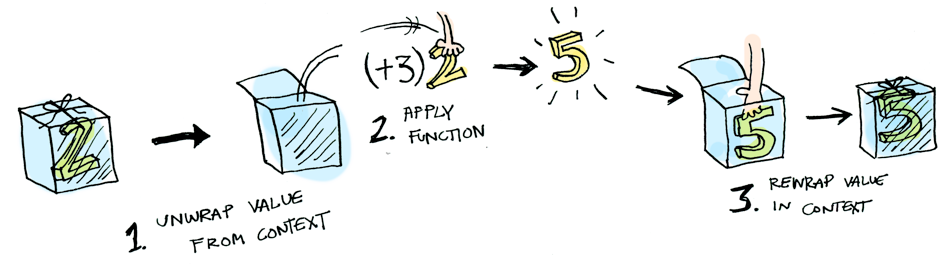Here’s what is happening behind the scenes when we try to map a function on an empty box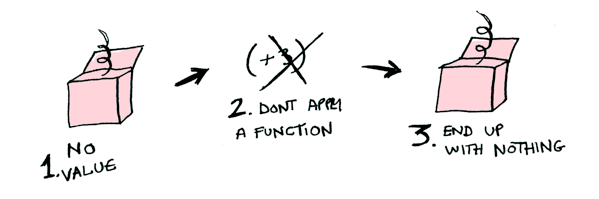Now, what happens when you apply a function to a list?``List.of(2, 4, 6).map(i -> i + 3); // (5, 7, 9)``

Functions are also functors!

In Java with vavr `map` is called `compose` or `andThen`

``````Function1<Integer, Integer> add3 = Function1.of(i -> i + 3);
Function1<Integer, Integer> add2 = Function1.of(i -> i + 2);

#### Applicatives

Applicatives are like functors, except that not only the value is being wrapped in a context, but the function to apply to it also.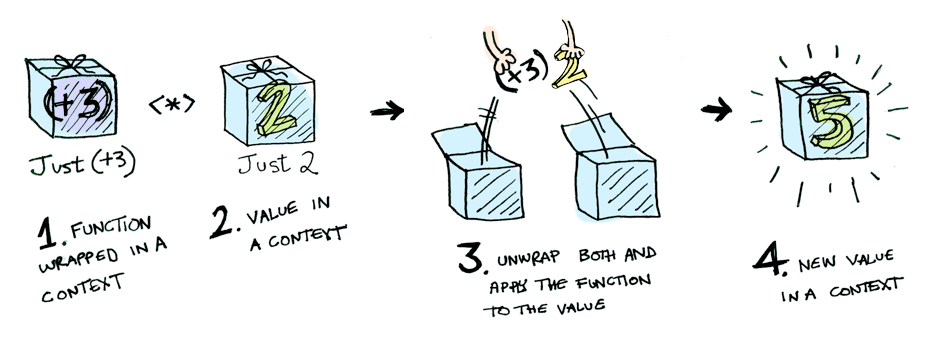Monads apply a function that returns a wrapped value to a wrapped value

Suppose a function named half which only works on even numbers:

``````Option<Double> half(Double x) {
return x % 2 == 0 ? Option.of(x / 2) : Option.none();
}``````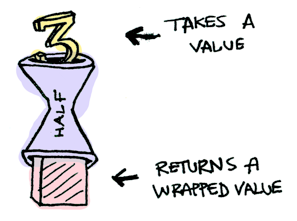But what if we feed it a wrapped value?This is where bind also called flatMap or sometimes chain comes in!

``````Option.of(3d).flatMap(this::half); // None
Option.of(4d).flatMap(this::half); // Some(2)``````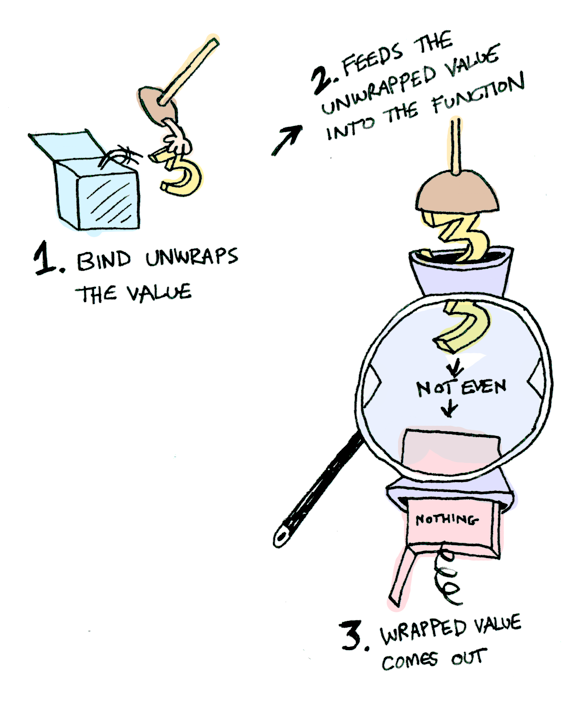If you pass in `None`, it’s even simpler: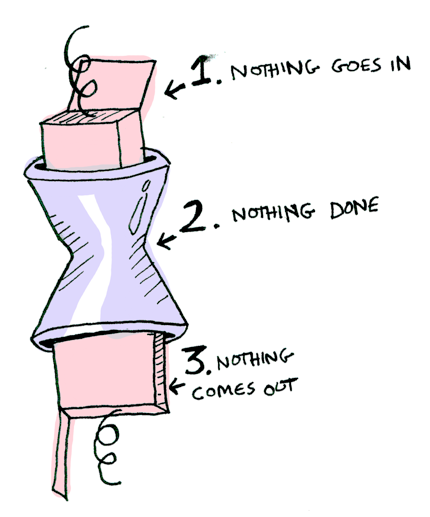You can chain calls to flatMap:

``````Option.of(20d)
.flatMap(this::half)  // Some(10)
.flatMap(this::half)  // Some(5)
.flatMap(this::half); // None``````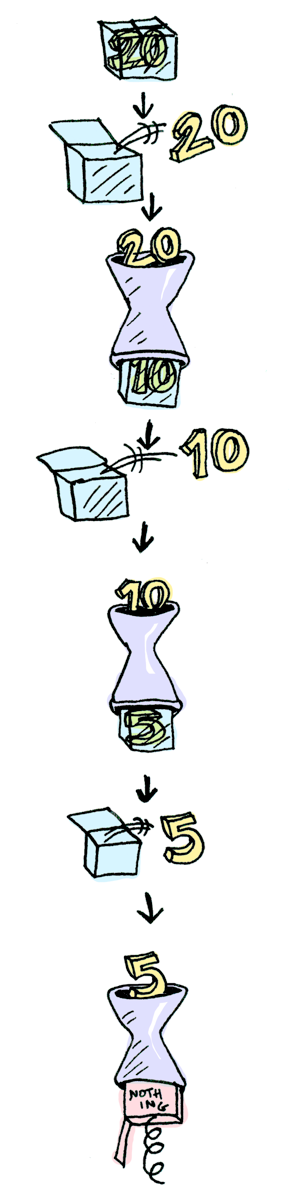So now we know that Option is a functor, an applicative and a monad.

Another example: user types a path, we load the file content and display it

``````Option<String> getLine() {
System.out.print("File: ");
return Option.of(new Scanner(System.in).next());
}

}

Option<Boolean> putStrLn(String str) {
System.out.println("File content:\n");
System.out.println(str);
return Option.of(str.length() % 2 == 0);
}``````And just call it like this:

``````getLine()
.flatMap(this::putStrLn);``````How to FP in Java with Vavr

• Functions
• Composition
• Lifting
• Partial application
• Currying
• Memoization
• Values
• Option
• Try
• Either

#### Functions

Java provides only:

• `Function`: accepts one parameter
• `BiFunction`: accepts two parameters

Vavr goes up to 8 parameters:

• `Function0`, `Function1`, `Function2`, …
• Support checked functions: `CheckedFunction1`, `CheckedFunction2`, …
• Support composition, lifting, currying and memoization
``````Function2<Integer, Integer, Integer> sum = (a, b) -> a + b;
sum.apply(40, 2); // 42``````
##### Composition

Functions can be composed:

• `f : X -> Y`
• `g : Y -Z`
• `h : X -> Z = g(f(x))`

Use `compose` or `andThen` for mor natural (for humans) order.

``````Function1<String, String> upperCase = Function1.of(String::toUpperCase);
Function1<String, String> hi = s -> "Hi, " + s;
Function1<String, String> addMark = s -> s + " !";

Function1<String, String> welcome =
welcome.apply("tars"); // Hi, TARS !

Function1<String, String> welcome2 =
welcome2.apply("tars"); // Hi, TARS !``````
##### Lifting

Partial Functions are functions that are valid for a specific subset of values but may yield errors for some input

The `lift` function lifts a partial function into a total function

• It can accept all input
• Returns an `Option` instead of the value
• Instead of throwing an exception it will return `None`
``````Function2<Integer, Integer, Integer> divide = (a, b) -> a / b;
Function2<Integer, Integer, Option<Integer>> safeDivide = Function2.lif(divide);

safeDivide.apply(15, 5); // Some(3)
safeDivide.apply(15, 0); // None``````
##### Partial application

Partial application allows you to create new function from an existing one by setting some arguments

It is not currying

``````Function2<Integer, Integer, Integer> multiply = (a, b) -> a * b;
Function1<Integer, Integer> twoTimes = multiply.apply(2);

multiply.apply(4, 3); // 12
twoTimes.apply(9); // 18

Function3<Long, Long, Long, Long> computation = (a, b, c) -> a + (b * c);
Function2<Long, Long, Long> incMultiply = computation.apply(1L);

incMultiply.apply(L2, 3L); // 1 + (2 * 3) = 7``````
##### Currying

Currying is the same as converting a function that takes n arguments into n functions taking a single argument each.

``````Function3<Long, Long, Long, Long> computation = (a, b, c) -> a + (b * c);
Function2<Long, Long, Long> incMultiply = computation.apply(1L);

incMultiply.apply(L2, 3L); // 1 + (2 * 3) = 7

Function1<Long, Function1<Long, Function1<Long, Long>>> curriedComputation =
computation.curried();

curriedComputation.apply(1L).apply(2L).apply(3L); // 1 + (2 * 3) = 7``````
##### Memoization

Memoization acts like some kind of caching, if you memoize a function, it will be only executed once for a specific input

``````Function0<String> cachedUUID = Function0.of(UUID::randomUUID)
.andThen(UUID::toString).memoized();

Function1<String, String> cachedUserUUID =
Function1.of((String user) -> user + ": " + UUID.randomUUID().toString())
.memoized();
cachedUserUUID.apply("Tars"); // Tars: d01562a7-1a13-45c0-b567-3239f4d0abc1
cachedUserUUID.apply("Kipp"); // Kipp: 304b1e2d-4c76-4785-9acc-de04ecf87730
cachedUserUUID.apply("Case"); // Case: 2e77448f-9a4d-4fc1-8b78-8805b035dd5b
cachedUserUUID.apply("Tars"); // Tars: d01562a7-1a13-45c0-b567-3239f4d0abc1``````

#### Values

##### Option

`Option` is a monadic container with additions:

• `map`
• `flatMap`
• `filter`
• `peek`

Represents an optional value: `None` / `Some(value)`.

``````final String[] robots = new String[] {"Tars", "Kipp", "Case"};
Function0<String> randomRobot = () -> {
Random r = new Random();
boolean shouldFail = r.nextInt(10) > 5;
return shouldFail ? null : robots[r.nextInt(3)];
};

Function1<String, String> upperCase = Function1.of(String::toUpperCase);
Option.of(randomRobot.apply()).map(upperCase); // None
Option.of(randomRobot.apply()).map(upperCase); // Some(KIPP)
Option.of(randomRobot.apply()).map(upperCase); // Some(TARS)
Option.of(randomRobot.apply()).map(upperCase); // Some(TARS)
Option.of(randomRobot.apply()).map(upperCase); // None
Option.of(randomRobot.apply()).map(upperCase); // Some(CASE)``````
##### Try

Try is a monadic container wich represents a computation that may either throw an exception of successfuly completes

Like `Option` it has a lot of additions:

• `map`
• `flatMap`
• `filter`
• `mapTry`
• `peek`
• `recover`
• `onFailure`
• `onSuccess`
``````final String[] robots = new String[] {"Tars", "Kipp", "Case"};
Function0<String> randomRobot = () -> {
Random r = new Random();
boolean shouldFail = r.nextInt(10) > 5;
if (shouldFail)
throw new RuntimeException("Plenty of slaves for my robot colony");
else
return robots[r.nextInt(3)];
};

Function1<String, String> upperCase = Function1.of(String::toUpperCase);
Try.of(randomRobot::apply).map(upperCase); // Failure(Plenty of slaves for my robot colony)
Try.of(randomRobot::apply).map(upperCase); // Some(CASE)
Try.of(randomRobot::apply).map(upperCase); // Some(KIPP)
Try.of(randomRobot::apply).map(upperCase); // Failure(Plenty of slaves for my robot colony)
Try.of(randomRobot::apply).map(upperCase); // Failure(Plenty of slaves for my robot colony)
Try.of(randomRobot::apply).map(upperCase); // Some(CASE)
Try.of(randomRobot::apply).map(upperCase); // Some(TARS)``````
##### Either

Either represents a value of two types, it is either a `Left` or a `Right`.

By convention `Left` is the failure case, and `Right` the success case, like `Option` and `Try` it has a lot of additions:

• `right`
• `left`
• `map`
• `flatMap`
• `filter`
``````Function0<Either<Throwable, String>> safeRandomRobot =
() -> {
Random r = new Random();
boolean shouldFail = r.nextInt(10) > 5;
return shouldFail ? Either.left(new RuntimeException("Plenty of slaves for my robot colony"))
: Either.right(robots[r.nextInt(3)]);
};

// Plenty of slaves for my robot colony
safeRandomRobot.apply().map(upperCase).getOrElsGet(Throwable::getMessage);
// TARS
safeRandomRobot.apply().map(upperCase).getOrElsGet(Throwable::getMessage);
// TARS
safeRandomRobot.apply().map(upperCase).getOrElsGet(Throwable::getMessage);
// CASE
safeRandomRobot.apply().map(upperCase).getOrElsGet(Throwable::getMessage);
// Plenty of slaves for my robot colony
safeRandomRobot.apply().map(upperCase).getOrElsGet(Throwable::getMessage);
// KIPP
safeRandomRobot.apply().map(upperCase).getOrElsGet(Throwable::getMessage);``````

That’s all for now, I encourage you to try Vavr, it can make your code both cleaner and safer at the same time.

Edit: See also my other article about how to use `Try` efficiently in the context of a pipeline.

Cheers!

Alexandre Grison - //grison.me - @algrison